﻿ Weight | Zona Land Education

# Weight

Weight is the pull of gravity.

An object placed near the surface of Earth experiences the pull downward of gravity. This pull, or force, is called the weight of the object. The weight of an object is the pull of gravity on that object.

Weight is a force.

Since weight is a force, it is measured in Newtons. A common symbol for it is:

# Fg

'F sub g' is often read 'the force of gravity' or 'the weight'.

Weight is a vector.

Since weight is a force and force is a vector, weight is a vector.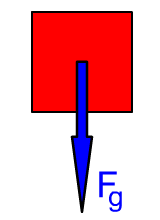Weight is what causes an object to fall.

The downward pull of the weight of an object is the force that causes the downward acceleration of that object. Objects fall because of their weight.

Without air resistance an object on Earth will accelerate at a rate of 9.8 m/s2 as it falls.

Normally, falling bodies experience air resistance. With air resistance, this acceleration would be decreased as the object fell. Under these conditions the object could reach a terminal velocity and speed up no more.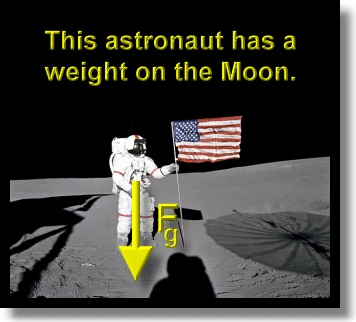Weight happens on any planet or moon.

An object would experience weight on any planet or moon. On the surface of the Earth's moon, for example, you would have a weight, you would feel the pull of the Moon's gravity.

Your weight on the Moon, though, would be one sixth of your weight on Earth. That is because the gravitational field strength on the Moon is much less than on Earth.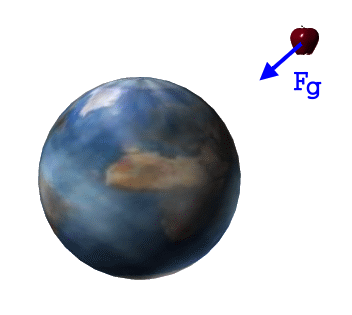The term weight usually refers to the force of gravity near the surface of a planet or a moon.

We usually use the word weight to refer to the pull of gravity near the surface of a planet. How near? Well, for practical purposes, you can use the word weight for the pull of gravity if you are close enough to the surface so that it appears flat to you. This would be true for locations at ground level up to a few miles high.

Certainly the object in the picture above is not near the surface of the Earth. It does still, though, feel the pull of gravity due to the Earth, even at this and greater distances. This is the same force of gravity as weight. However, in situations like this the force of gravity is usually not called the weight of the object. It is simply referred to as the gravitational force on the object. It is a vector, and it is also normally symbolized with Fg.

Formula for weightAcceleration  due to gravity

When an object falls, it is pulled down by the force of gravity. This constant force, called the weight, causes the object to accelerate as it moves downward. This acceleration is the same for all bodies (all bodies on the same planet or moon), and it is called the acceleration due to gravity.

The acceleration due to gravity (on Earth) has a size of 9.8 m/s2. The symbol ag is often used for this acceleration, so we have: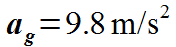Lower case g is also often used for this acceleration, so we also have: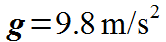Derive the weight formula from Newton's Second Law of Motion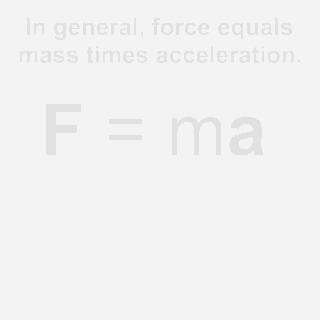We will use this acceleration due to gravity to make our formula for weight. First, we note that according to Newton's Second Law of Motion, the force on an object equals its mass times its acceleration, or: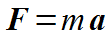Since our force is the weight of the object, Fg, and our acceleration is ag, we can write this formula as: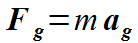Or, using g for the acceleration due to gravity, we could write: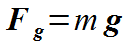'The weight of an object equals the object's mass times the acceleration due to gravity.'

Since weight is the force of gravity, you could also read these equations as:

'The force of gravity on an object equals the object's mass times the acceleration due to gravity.'

Example problems

On Earth

What is the weight of an object with a mass of 2.5 kg on planet Earth? Here is that calculation: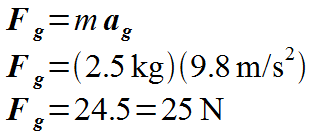An object with a mass of 2.5 kg weighs 25 N on Earth. Note that mass and weight are different concepts.

On the Moon

What about on the Moon? On the Moon the acceleration due to gravity is different than it is on Earth. On the Moon ag is 1.6 m/s2. If this 2.5 kg object were taken to the Moon, it would have a different weight than it has on Earth. Here is the calculation for the weight on the Moon: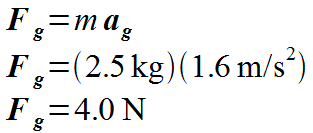This 2.5 kg object weighs 4.0 N on the Moon.

Mass is not weight.

Note in the above two problems that the mass of the object is constant; it is 2.5 kg both on the Earth and on the Moon. However, the object weighs more on the Earth than it weighs on the Moon. This is because the gravitational field near the Earth's surface is greater in strength than the gravitational field near the Moon's surface.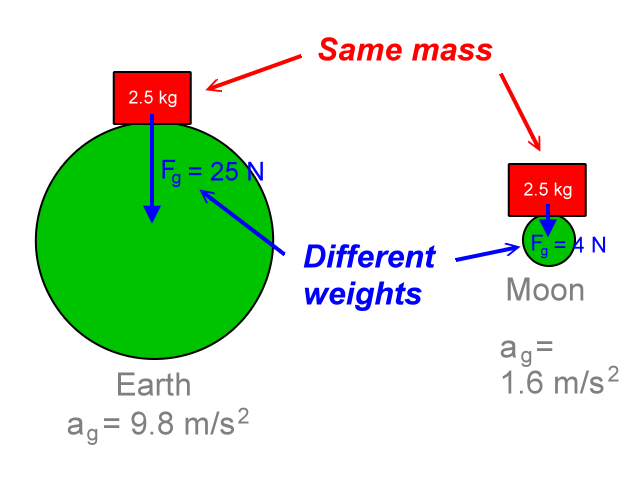The Earth's stronger gravitational field pulls harder on 2.5 kg than the Moon's weaker gravitational field pulls on 2.5 kg.  The stronger force on Earth causes 2.5 kg to have a greater acceleration while falling than the weaker force on the Moon causes for the same 2.5 kg.

Be sure to notice that if the object were taken from the Earth to the Moon, its mass would stay the same, but its weight would change. Weight and mass are related, but different ideas.